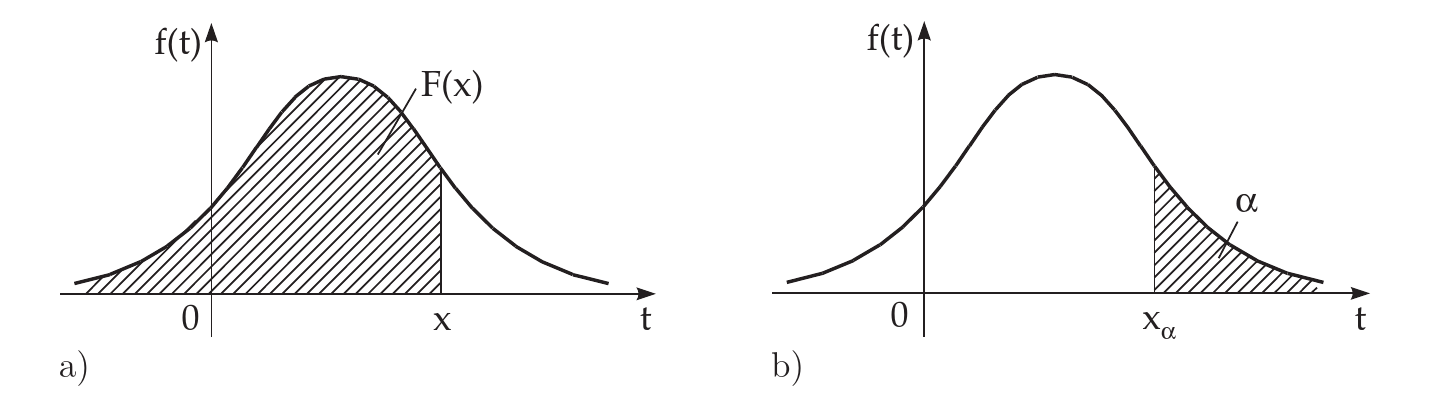This is part of the mo­ments math col­lec­tion.

## Random Variables

For a space of el­e­men­tary events, say \Omega=\{\omega\}, a ran­dom vari­able X is a real num­ber func­tion X=X(\omega) de­ﬁned on the set \Omega.

Essentially, X may be con­sid­ered to be a quan­tity which takes its val­ues (say x_i) from a sub­set R of real num­bers.

We note that iff X is a ran­dom vari­able, a func­tion g(X) is also ran­dom.

Random vari­ables are fur­ther quan­ti­ﬁed and clas­si­ﬁed on the ba­sis of their dis­tri­b­u­tion func­tions.

Distribution Law

A rule (tabular, func­tional, graph­i­cal, etc) which per­mits one to ﬁnd the prob­a­bil­i­ties of an event (a.k.a the ran­dom vari­able) is the dis­tri­b­u­tion law for the ran­dom vari­able.

## Distribution Functions

Every ran­dom vari­able is de­ﬁned in terms of it’s prob­a­bil­i­ties, i.e they are char­ac­ter­ized by the like­li­hood of hav­ing a par­tic­u­lar value.

Mathematically, the cu­mu­la­tive dis­tri­b­u­tion func­tion of a ran­dom vari­able X is the func­tion F(x) whose value at every point x is equal to the prob­a­bil­ity of the event {X <x}:

F(x)=P(X<x)

### Properties

• 0 \leq F(x) \leq 1
• \lim_{x\to -\infty}F(x)=F(-\infty)=0 and \lim_{x\to\infty}F(x)=F(\infty)=1
• \forall x_i, x_2>x_1 \implies F(x_2)\geq F(x1)
• P(x_1 \leq X < x_2)=F(x_2)-F(x_1)
• F(x) is left con­tin­u­ous. (i.e., \lim_{x\to(x_0-0)}F(x)=F(x_0))

## Types of RandomVariables

On the ba­sis of the above con­cepts, we now quan­tify ran­dom vari­ables as: X \to (1)

## Expectation

The ex­pec­ta­tion (expected value) E(X) of a dis­crete or con­tin­u­ous ran­dom vari­able X is math­e­mat­i­cally de­ﬁned by: E\{X\}= (2)

For the con­tin­u­ous case, it is nec­es­sary that the in­te­gral or it’s cor­re­spond­ing se­ries con­verges ab­solutely.

In generic terms, the ex­pec­ta­tion is the main char­ac­ter­is­tic deﬁn­ing the position” of a ran­dom vari­able, i.e., the num­ber near which its pos­si­ble val­ues are con­cen­trated.

Similarly, due to the sim­i­lar­ity of func­tions de­scrib­ing ran­dom vari­ables and ran­dom vari­ables, given a ran­dom vari­able Y re­lated to a ran­dom vari­able X by a func­tional de­pen­dence Y=\phi(X) then we have:

E\{Y\}=E\{\phi(X)\}= (3)

## Variance

The vari­ance, Var{X} is the mea­sure of de­vi­a­tion of a ran­dom vari­able X from the ex­pec­ta­tion E\{X\} as de­ter­mined by:

\text{Var}\{X\}=E\{(X-E\{X\})^2\} (4)

The vari­ance char­ac­ter­izes the spread in val­ues of the ran­dom vari­able X about its ex­pec­ta­tion.

## Graphical Preliminaries

Having in­tro­duced the den­sity func­tion and the dis­tri­b­u­tion func­tion, it is triv­ial to in­ter­pret the fol­low­ing curves in the ﬁg­ure be­low and note, that the prob­a­bil­ity P(X\leq x)=F(x) may be rep­re­sented as an area be­tween the den­sity func­tion f(t) and the x-axis on the in­ter­val -\infty<t\leq xProbability as an area.1

Often there is given (frequently in %) a prob­a­bil­ity value \alpha.

If P(X > x) = \alpha holds, the cor­re­spond­ing value of the ab­scissa x = x_\alpha is called the quan­tile or the frac­tile of or­der \alpha

This means the area un­der the den­sity func­tion f(t) to the right of x = x_\alpha is equal to \alpha.

Remark: In the lit­er­a­ture, the area to the left of x = x_\alpha is also used for the de­f­i­n­i­tion of quan­tile.

In math­e­mat­i­cal sta­tis­tics, for small val­ues of \alpha, e.g., \alpha= 5\% or \alpha= 1\%, is also used the no­tion sig­nif­i­cance level of ﬁrst type or type 1 er­ror rate.

Bronshtein, I.N., K.A. Semendyayev, G. Musiol, and H. Mühlig. 2015. Handbook of Mathematics. Springer Berlin Heidelberg. https://​books.google.co.in/​books?id=5L6BB­wAAQBAJ.

1. Bronshtein et al. (2015)